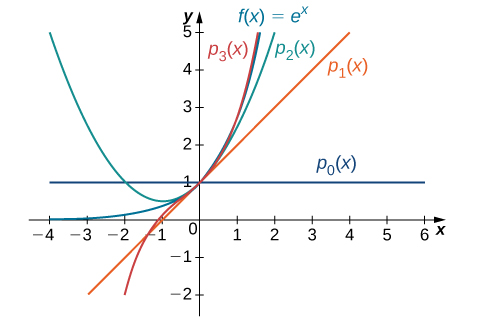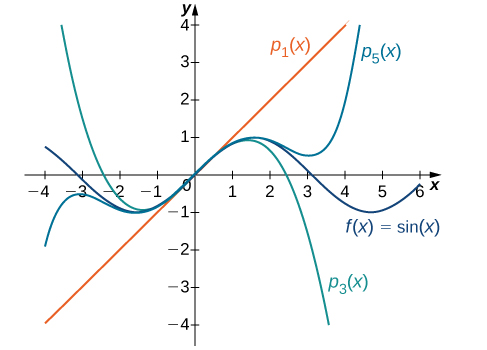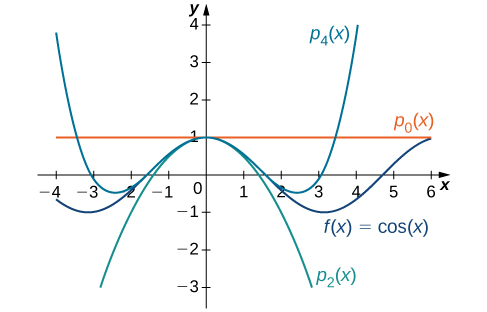# 6.3 Taylor and maclaurin series  (Page 2/13)

 Page 2 / 13

## Uniqueness of taylor series

If a function $f$ has a power series at a that converges to $f$ on some open interval containing a , then that power series is the Taylor series for $f$ at a .

The proof follows directly from [link] .

To determine if a Taylor series converges, we need to look at its sequence of partial sums. These partial sums are finite polynomials, known as Taylor polynomials    .

Visit the MacTutor History of Mathematics archive to read brief biographies of Brook Taylor and Colin Maclaurin and how they developed the concepts named after them.

## Taylor polynomials

The n th partial sum of the Taylor series for a function $f$ at $a$ is known as the n th Taylor polynomial. For example, the 0th, 1st, 2nd, and 3rd partial sums of the Taylor series are given by

$\begin{array}{c}{p}_{0}\left(x\right)=f\left(a\right),\hfill \\ {p}_{1}\left(x\right)=f\left(a\right)+{f}^{\prime }\left(a\right)\left(x-a\right),\hfill \\ {p}_{2}\left(x\right)=f\left(a\right)+{f}^{\prime }\left(a\right)\left(x-a\right)+\frac{f\text{″}\left(a\right)}{2\text{!}}{\left(x-a\right)}^{2},\hfill \\ {p}_{3}\left(x\right)=f\left(a\right)+{f}^{\prime }\left(a\right)\left(x-a\right)+\frac{f\text{″}\left(a\right)}{2\text{!}}{\left(x-a\right)}^{2}+\frac{f\text{‴}\left(a\right)}{3\text{!}}{\left(x-a\right)}^{3},\hfill \end{array}$

respectively. These partial sums are known as the 0th, 1st, 2nd, and 3rd Taylor polynomials of $f$ at $a,$ respectively. If $x=a,$ then these polynomials are known as Maclaurin polynomials for $f.$ We now provide a formal definition of Taylor and Maclaurin polynomials for a function $f.$

## Definition

If $f$ has n derivatives at $x=a,$ then the n th Taylor polynomial for $f$ at $a$ is

${p}_{n}\left(x\right)=f\left(a\right)+{f}^{\prime }\left(a\right)\left(x-a\right)+\frac{f\text{″}\left(a\right)}{2\text{!}}{\left(x-a\right)}^{2}+\frac{f\text{‴}\left(a\right)}{3\text{!}}{\left(x-a\right)}^{3}+\text{⋯}+\frac{{f}^{\left(n\right)}\left(a\right)}{n\text{!}}{\left(x-a\right)}^{n}.$

The n th Taylor polynomial for $f$ at 0 is known as the n th Maclaurin polynomial for $f.$

We now show how to use this definition to find several Taylor polynomials for $f\left(x\right)=\text{ln}\phantom{\rule{0.1em}{0ex}}x$ at $x=1.$

## Finding taylor polynomials

Find the Taylor polynomials ${p}_{0},{p}_{1},{p}_{2}$ and ${p}_{3}$ for $f\left(x\right)=\text{ln}\phantom{\rule{0.1em}{0ex}}x$ at $x=1.$ Use a graphing utility to compare the graph of $f$ with the graphs of ${p}_{0},{p}_{1},{p}_{2}$ and ${p}_{3}.$

To find these Taylor polynomials, we need to evaluate $f$ and its first three derivatives at $x=1.$

$\begin{array}{cccccccc}\hfill f\left(x\right)& =\hfill & \text{ln}\phantom{\rule{0.1em}{0ex}}x\hfill & & & \hfill f\left(1\right)& =\hfill & 0\hfill \\ \hfill {f}^{\prime }\left(x\right)& =\hfill & \frac{1}{x}\hfill & & & \hfill {f}^{\prime }\left(1\right)& =\hfill & 1\hfill \\ \hfill f\text{″}\left(x\right)& =\hfill & -\frac{1}{{x}^{2}}\hfill & & & \hfill f\text{″}\left(1\right)& =\hfill & -1\hfill \\ \hfill f\text{‴}\left(x\right)& =\hfill & \frac{2}{{x}^{3}}\hfill & & & \hfill f\text{‴}\left(1\right)& =\hfill & 2\hfill \end{array}$

Therefore,

$\begin{array}{ccc}\hfill {p}_{0}\left(x\right)& =\hfill & f\left(1\right)=0,\hfill \\ \hfill {p}_{1}\left(x\right)& =\hfill & f\left(1\right)+{f}^{\prime }\left(1\right)\left(x-1\right)=x-1,\hfill \\ \hfill {p}_{2}\left(x\right)& =\hfill & f\left(1\right)+{f}^{\prime }\left(1\right)\left(x-1\right)+\frac{f\text{″}\left(1\right)}{2}{\left(x-1\right)}^{2}=\left(x-1\right)-\frac{1}{2}{\left(x-1\right)}^{2},\hfill \\ \hfill {p}_{3}\left(x\right)& =\hfill & f\left(1\right)+{f}^{\prime }\left(1\right)\left(x-1\right)+\frac{f\text{″}\left(1\right)}{2}{\left(x-1\right)}^{2}+\frac{f\text{‴}\left(1\right)}{3\text{!}}{\left(x-1\right)}^{3}\hfill \\ & =\hfill & \left(x-1\right)-\frac{1}{2}{\left(x-1\right)}^{2}+\frac{1}{3}{\left(x-1\right)}^{3}.\hfill \end{array}$

The graphs of $y=f\left(x\right)$ and the first three Taylor polynomials are shown in [link] .The function y = ln x and the Taylor polynomials p 0 , p 1 , p 2 and p 3 at x = 1 are plotted on this graph.

Find the Taylor polynomials ${p}_{0},{p}_{1},{p}_{2}$ and ${p}_{3}$ for $f\left(x\right)=\frac{1}{{x}^{2}}$ at $x=1.$

${p}_{0}\left(x\right)=1;{p}_{1}\left(x\right)=1-2\left(x-1\right);{p}_{2}\left(x\right)=1-2\left(x-1\right)+3{\left(x-1\right)}^{2};{p}_{3}\left(x\right)=1-2\left(x-1\right)+3{\left(x-1\right)}^{2}-4{\left(x-1\right)}^{3}$

We now show how to find Maclaurin polynomials for e x , $\text{sin}\phantom{\rule{0.1em}{0ex}}x,$ and $\text{cos}\phantom{\rule{0.1em}{0ex}}x.$ As stated above, Maclaurin polynomials are Taylor polynomials centered at zero.

## Finding maclaurin polynomials

For each of the following functions, find formulas for the Maclaurin polynomials ${p}_{0},{p}_{1},{p}_{2}$ and ${p}_{3}.$ Find a formula for the n th Maclaurin polynomial and write it using sigma notation. Use a graphing utilty to compare the graphs of ${p}_{0},{p}_{1},{p}_{2}$ and ${p}_{3}$ with $f.$

1. $f\left(x\right)={e}^{x}$
2. $f\left(x\right)=\text{sin}\phantom{\rule{0.1em}{0ex}}x$
3. $f\left(x\right)=\text{cos}\phantom{\rule{0.1em}{0ex}}x$
1. Since $f\left(x\right)={e}^{x},$ we know that $f\left(x\right)={f}^{\prime }\left(x\right)=f\text{″}\left(x\right)=\text{⋯}={f}^{\left(n\right)}\left(x\right)={e}^{x}$ for all positive integers n . Therefore,
$f\left(0\right)={f}^{\prime }\left(0\right)=f\text{″}\left(0\right)=\text{⋯}={f}^{\left(n\right)}\left(0\right)=1$

for all positive integers n . Therefore, we have
$\begin{array}{ccc}\hfill {p}_{0}\left(x\right)& =\hfill & f\left(0\right)=1,\hfill \\ \hfill {p}_{1}\left(x\right)& =\hfill & f\left(0\right)+{f}^{\prime }\left(0\right)x=1+x,\hfill \\ \hfill {p}_{2}\left(x\right)& =\hfill & f\left(0\right)+{f}^{\prime }\left(0\right)x+\frac{f\text{″}\left(0\right)}{2\text{!}}{x}^{2}=1+x+\frac{1}{2}{x}^{2},\hfill \\ \hfill {p}_{3}\left(x\right)& =\hfill & f\left(0\right)+{f}^{\prime }\left(0\right)x+\frac{f\text{″}\left(0\right)}{2}{x}^{2}+\frac{f\text{‴}\left(0\right)}{3\text{!}}{x}^{3}\hfill \\ & =\hfill & 1+x+\frac{1}{2}{x}^{2}+\frac{1}{3\text{!}}{x}^{3},\hfill \\ \hfill {p}_{n}\left(x\right)& =\hfill & f\left(0\right)+{f}^{\prime }\left(0\right)x+\frac{f\text{″}\left(0\right)}{2}{x}^{2}+\frac{f\text{‴}\left(0\right)}{3\text{!}}{x}^{3}+\text{⋯}+\frac{{f}^{\left(n\right)}\left(0\right)}{n\text{!}}{x}^{n}\hfill \\ & =\hfill & 1+x+\frac{{x}^{2}}{2\text{!}}+\frac{{x}^{3}}{3\text{!}}+\text{⋯}+\frac{{x}^{n}}{n\text{!}}\hfill \\ & =\hfill & \sum _{k=0}^{n}\frac{{x}^{k}}{k\text{!}}.\hfill \end{array}$

The function and the first three Maclaurin polynomials are shown in [link] .The graph shows the function y = e x and the Maclaurin polynomials p 0 , p 1 , p 2 and p 3 .
2. For $f\left(x\right)=\text{sin}\phantom{\rule{0.1em}{0ex}}x,$ the values of the function and its first four derivatives at $x=0$ are given as follows:
$\begin{array}{cccccccc}\hfill f\left(x\right)& =\hfill & \text{sin}\phantom{\rule{0.1em}{0ex}}x\hfill & & & \hfill f\left(0\right)& =\hfill & 0\hfill \\ \hfill {f}^{\prime }\left(x\right)& =\hfill & \text{cos}\phantom{\rule{0.1em}{0ex}}x\hfill & & & \hfill {f}^{\prime }\left(0\right)& =\hfill & 1\hfill \\ \hfill f\text{″}\left(x\right)& =\hfill & \text{−}\text{sin}\phantom{\rule{0.1em}{0ex}}x\hfill & & & \hfill f\text{″}\left(0\right)& =\hfill & 0\hfill \\ \hfill f\text{‴}\left(x\right)& =\hfill & \text{−}\text{cos}\phantom{\rule{0.1em}{0ex}}x\hfill & & & \hfill f\text{‴}\left(0\right)& =\hfill & -1\hfill \\ \hfill {f}^{\left(4\right)}\left(x\right)& =\hfill & \text{sin}\phantom{\rule{0.1em}{0ex}}x\hfill & & & \hfill {f}^{\left(4\right)}\left(0\right)& =\hfill & 0.\hfill \end{array}$

Since the fourth derivative is $\text{sin}\phantom{\rule{0.1em}{0ex}}x,$ the pattern repeats. That is, ${f}^{\left(2m\right)}\left(0\right)=0$ and ${f}^{\left(2m+1\right)}\left(0\right)={\left(-1\right)}^{m}$ for $m\ge 0.$ Thus, we have
$\begin{array}{c}{p}_{0}\left(x\right)=0,\hfill \\ {p}_{1}\left(x\right)=0+x=x,\hfill \\ {p}_{2}\left(x\right)=0+x+0=x,\hfill \\ {p}_{3}\left(x\right)=0+x+0-\frac{1}{3\text{!}}{x}^{3}=x-\frac{{x}^{3}}{3\text{!}},\hfill \\ {p}_{4}\left(x\right)=0+x+0-\frac{1}{3\text{!}}{x}^{3}+0=x-\frac{{x}^{3}}{3\text{!}},\hfill \\ {p}_{5}\left(x\right)=0+x+0-\frac{1}{3\text{!}}{x}^{3}+0+\frac{1}{5\text{!}}{x}^{5}=x-\frac{{x}^{3}}{3\text{!}}+\frac{{x}^{5}}{5\text{!}},\hfill \end{array}$

and for $m\ge 0,$
$\begin{array}{cc}\hfill {p}_{2m+1}\left(x\right)& ={p}_{2m+2}\left(x\right)\hfill \\ & =x-\frac{{x}^{3}}{3\text{!}}+\frac{{x}^{5}}{5\text{!}}-\text{⋯}+{\left(-1\right)}^{m}\frac{{x}^{2m+1}}{\left(2m+1\right)\text{!}}\hfill \\ & =\sum _{k=0}^{m}{\left(-1\right)}^{k}\frac{{x}^{2k+1}}{\left(2k+1\right)\text{!}}.\hfill \end{array}$

Graphs of the function and its Maclaurin polynomials are shown in [link] .The graph shows the function y = sin x and the Maclaurin polynomials p 1 , p 3 and p 5 .
3. For $f\left(x\right)=\text{cos}\phantom{\rule{0.1em}{0ex}}x,$ the values of the function and its first four derivatives at $x=0$ are given as follows:
$\begin{array}{cccccccc}\hfill f\left(x\right)& =\hfill & \text{cos}\phantom{\rule{0.1em}{0ex}}x\hfill & & & \hfill f\left(0\right)& =\hfill & 1\hfill \\ \hfill {f}^{\prime }\left(x\right)& =\hfill & \text{−}\text{sin}\phantom{\rule{0.1em}{0ex}}x\hfill & & & \hfill {f}^{\prime }\left(0\right)& =\hfill & 0\hfill \\ \hfill f\text{″}\left(x\right)& =\hfill & \text{−}\text{cos}\phantom{\rule{0.1em}{0ex}}x\hfill & & & \hfill f\text{″}\left(0\right)& =\hfill & -1\hfill \\ \hfill f\text{‴}\left(x\right)& =\hfill & \text{sin}\phantom{\rule{0.1em}{0ex}}x\hfill & & & \hfill f\text{‴}\left(0\right)& =\hfill & 0\hfill \\ \hfill {f}^{\left(4\right)}\left(x\right)& =\hfill & \text{cos}\phantom{\rule{0.1em}{0ex}}x\hfill & & & \hfill {f}^{\left(4\right)}\left(0\right)& =\hfill & 1.\hfill \end{array}$

Since the fourth derivative is $\text{sin}\phantom{\rule{0.1em}{0ex}}x,$ the pattern repeats. In other words, ${f}^{\left(2m\right)}\left(0\right)={\left(-1\right)}^{m}$ and ${f}^{\left(2m+1\right)}=0$ for $m\ge 0.$ Therefore,
$\begin{array}{c}{p}_{0}\left(x\right)=1,\hfill \\ {p}_{1}\left(x\right)=1+0=1,\hfill \\ {p}_{2}\left(x\right)=1+0-\frac{1}{2\text{!}}{x}^{2}=1-\frac{{x}^{2}}{2\text{!}},\hfill \\ {p}_{3}\left(x\right)=1+0-\frac{1}{2\text{!}}{x}^{2}+0=1-\frac{{x}^{2}}{2\text{!}},\hfill \\ {p}_{4}\left(x\right)=1+0-\frac{1}{2\text{!}}{x}^{2}+0+\frac{1}{4\text{!}}{x}^{4}=1-\frac{{x}^{2}}{2\text{!}}+\frac{{x}^{4}}{4\text{!}},\hfill \\ {p}_{5}\left(x\right)=1+0-\frac{1}{2\text{!}}{x}^{2}+0+\frac{1}{4\text{!}}{x}^{4}+0=1-\frac{{x}^{2}}{2\text{!}}+\frac{{x}^{4}}{4\text{!}},\hfill \end{array}$

and for $n\ge 0,$
$\begin{array}{cc}\hfill {p}_{2m}\left(x\right)& ={p}_{2m+1}\left(x\right)\hfill \\ & =1-\frac{{x}^{2}}{2\text{!}}+\frac{{x}^{4}}{4\text{!}}-\text{⋯}+{\left(-1\right)}^{m}\frac{{x}^{2m}}{\left(2m\right)\text{!}}\hfill \\ & =\sum _{k=0}^{m}{\left(-1\right)}^{k}\frac{{x}^{2k}}{\left(2k\right)\text{!}}.\hfill \end{array}$

Graphs of the function and the Maclaurin polynomials appear in [link] .The function y = cos x and the Maclaurin polynomials p 0 , p 2 and p 4 are plotted on this graph.

how can chip be made from sand
are nano particles real
yeah
Joseph
Hello, if I study Physics teacher in bachelor, can I study Nanotechnology in master?
no can't
Lohitha
where we get a research paper on Nano chemistry....?
nanopartical of organic/inorganic / physical chemistry , pdf / thesis / review
Ali
what are the products of Nano chemistry?
There are lots of products of nano chemistry... Like nano coatings.....carbon fiber.. And lots of others..
learn
Even nanotechnology is pretty much all about chemistry... Its the chemistry on quantum or atomic level
learn
da
no nanotechnology is also a part of physics and maths it requires angle formulas and some pressure regarding concepts
Bhagvanji
hey
Giriraj
Preparation and Applications of Nanomaterial for Drug Delivery
revolt
da
Application of nanotechnology in medicine
has a lot of application modern world
Kamaluddeen
yes
narayan
what is variations in raman spectra for nanomaterials
ya I also want to know the raman spectra
Bhagvanji
I only see partial conversation and what's the question here!
what about nanotechnology for water purification
please someone correct me if I'm wrong but I think one can use nanoparticles, specially silver nanoparticles for water treatment.
Damian
yes that's correct
Professor
I think
Professor
Nasa has use it in the 60's, copper as water purification in the moon travel.
Alexandre
nanocopper obvius
Alexandre
what is the stm
is there industrial application of fullrenes. What is the method to prepare fullrene on large scale.?
Rafiq
industrial application...? mmm I think on the medical side as drug carrier, but you should go deeper on your research, I may be wrong
Damian
How we are making nano material?
what is a peer
What is meant by 'nano scale'?
What is STMs full form?
LITNING
scanning tunneling microscope
Sahil
how nano science is used for hydrophobicity
Santosh
Do u think that Graphene and Fullrene fiber can be used to make Air Plane body structure the lightest and strongest. Rafiq
Rafiq
what is differents between GO and RGO?
Mahi
what is simplest way to understand the applications of nano robots used to detect the cancer affected cell of human body.? How this robot is carried to required site of body cell.? what will be the carrier material and how can be detected that correct delivery of drug is done Rafiq
Rafiq
if virus is killing to make ARTIFICIAL DNA OF GRAPHENE FOR KILLED THE VIRUS .THIS IS OUR ASSUMPTION
Anam
analytical skills graphene is prepared to kill any type viruses .
Anam
Any one who tell me about Preparation and application of Nanomaterial for drug Delivery
Hafiz
what is Nano technology ?
write examples of Nano molecule?
Bob
The nanotechnology is as new science, to scale nanometric
brayan
nanotechnology is the study, desing, synthesis, manipulation and application of materials and functional systems through control of matter at nanoscale
Damian
how did you get the value of 2000N.What calculations are needed to arrive at it
Privacy Information Security Software Version 1.1a
Good
Leaves accumulate on the forest floor at a rate of 2 g/cm2/yr and also decompose at a rate of 90% per year. Write a differential equation governing the number of grams of leaf litter per square centimeter of forest floor, assuming at time 0 there is no leaf litter on the ground. Does this amount approach a steady value? What is that value?
You have a cup of coffee at temperature 70°C, which you let cool 10 minutes before you pour in the same amount of milk at 1°C as in the preceding problem. How does the temperature compare to the previous cup after 10 minutes?
Abdul

#### Get Jobilize Job Search Mobile App in your pocket Now!By Yacoub JayoghliBy OpenStaxBy OpenStaxBy OpenStaxBy OpenStaxBy OpenStaxBy John GabrieliBy Sarah WarrenBy Madison ChristianBy Stephen Voron